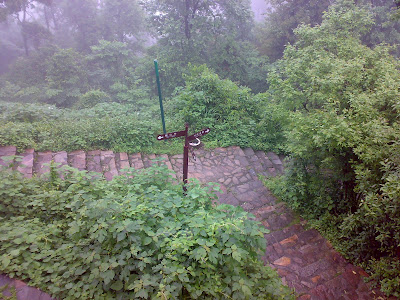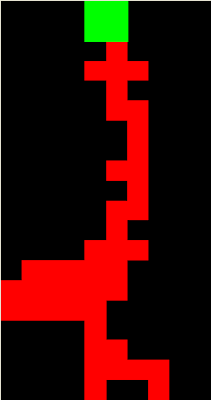```欢迎访问infoheader的Blog——年华似水浪淘沙
```

## 2009年5月12日星期二

### 祭一年前的这一天。

大约14点28分。我不确认，但不到30分，因为还没有上课。

我在教室里看语文书。一阵头晕。然后意识到了大地的晃动。

随后应急开始，学生到操场集合。

然后等待……直到确切的消息传来。

没有预料竟然是汶川。传了这么远。

512这一天，是民族的灾难。

然而没有灾难的民族是不幸的。

人们在灾难中学会坚强。

愿逝者安息。

生者前行。

## 2009年5月4日星期一

### 随想

开学已经三个月了。似乎没有什么特别的。无外乎早起上课，自习，偶尔无聊一下，然后睡觉，日复一日。明天是五月四日，青年节。觉得自己应该有点儿激情，才符合青年的特征。然而也许并没有。因为我喜欢平静地看待这个世界。已经开始渐渐习惯这样的生活。

用硬纸盒给自己做了一个书架，摆了几本书，放在床头。把小桌板和能充电的小灯找了出来。望自己能习惯看书。既然无法避免浅薄与无知，我希望书能改变自己。

偶尔有些思念。想着七月的见面。不知道那时又会怎样。我希望见到我要的结局。人可以不聪明，但是一定要有思想。我希望他们得到智慧。

五一那天帮同学选了一台笔记本电脑，同学很满意。但是感到自己距离计算机越来越远了。或许这不是一件坏事。毕竟学的专业不是计算机科学。路是自己走出来的。我还没有真正看到电子专业是怎样的，所以我不知道在这个岔口自己会怎样选择。

外面有蟋蟀，有远方的火车，还有一个凉夜，一切是如此的熟悉，就像在家，也像在高中租的房子。真的很像。我想起我经常透过窗子，看到自己的眼睛。现在我似乎也可以看到了，穿越黑暗，它依然在望着什么，和以前一样明亮。

## 2009年5月1日星期五

### 昨天的行程

于清晨游岳麓山，游则尽兴，尽兴而归。有雨。怀古。## 2009年4月28日星期二

### J2ME

`import javax.microedition.midlet.*;import javax.microedition.lcdui.*;public class HelloWorld extends MIDlet implements CommandListener{ private Display ds; private Command exitCom; private TextBox tb;  public HelloWorld() {  ds=Display.getDisplay(this);  exitCom=new Command("Exit",Command.EXIT,1);  tb=new TextBox("Hello MIDlet","Hello,World!",15,0);  tb.addCommand(exitCom);  tb.setCommandListener(this); }  protected void startApp(){  ds.setCurrent(tb); }  protected void pauseApp(){ }  protected void destroyApp(boolean u){ }  public void commandAction(Command c,Displayable d){  if(c==exitCom){   destroyApp(false);   notifyDestroyed();  } }}`

## 2009年4月27日星期一

### 无题

忙碌了这么长时间，终于可以静下心来写一篇日志了。

回到寝室的反应就是累，困。屋里也没有人，不知不觉就睡着了。直到外面隐隐约约的笛子声响起，不知道师大音乐系的宿舍里又有谁在练习了。一时见有种莫名的感动。那旋律好熟悉，竟然想不起名字。可能是我淡忘了，我如此喜欢的班得瑞的《童年》。

有六天的休息时间，然后是金工实习，又要忙碌了。

## 2009年4月14日星期二

### 最近的生活

课很多，以至于没有什么时间做其他的事情。每天都是上课，看书，写作业，睡觉。
清明节那天去了一次韶山。虽然天气不好，但是玩的还算高兴。看了刘少奇故居，纪念馆，毛泽东故居，韶山山顶等等。刘少奇故居

前几个星期一直在下雨，我觉得这雨断断续续有一个多月了。这两天总算是晴朗了一下。

## 2009年3月31日星期二

### VB俄罗斯方块1. Const WidthCount As Integer = 10

2. Const HeightCount As Integer = 20

3. '"ttttffffffffffff"

4. '"ttffttffffffffff"

5. '"tttfftffffffffff"

6. '"ttfffttfffffffff"

7. '"tttftftfffffffff"

8. 'Dim BlockS(1 To 10) As String

9. Const BlockSCount As Integer = 8

10. Dim Block(1 To 10, 1 To 4, 1 To 4, 1 To 4) As Boolean

11. Dim P As PictureBox

12. Dim B() As Integer

13. Dim PosX As Integer

14. Dim PosY As Integer

15. Dim CurrentBlock As Integer

16. Dim CurrentTurn As Integer

17. Private Sub Form_KeyDown(KeyCode As Integer, Shift As Integer)

18.     If KeyCode = vbKeyReturn Then

19.         ReStart

20.         Exit Sub

21.     End If

22.     If Timer1.Enabled Then

23.         If KeyCode = vbKeyLeft And PosX > 0 Then

24.             If Not CheckSet(PosX - 1, PosY) Then

25.                 PosX = PosX - 1

26.             End If

27.         ElseIf KeyCode = vbKeyRight And PosX < WidthCount - GetBlockWidth(CurrentBlock) Then

28.             If Not CheckSet(PosX + 1, PosY) Then

29.                 PosX = PosX + 1

30.             End If

31.         ElseIf KeyCode = vbKeyUp Then

32.             TurnBlock

33.         ElseIf KeyCode = vbKeyDown Then

34.     '        Do

35.     '            If CheckSet(PosX, PosY + 1) Then

36.     '                SetBlock

37.     '                CheckDecrease

38.     '                NextBlock

39.     '                Exit Do

40.     '            Else

41.     '                PosY = PosY + 1

42.     '                Draw

43.     '            End If

44.     '        Loop

45.             Timer1_Timer

46.             Timer1.Interval = 20

47.         End If

48.         Draw

49.     End If

50. End Sub

51. Private Sub Form_KeyUp(KeyCode As Integer, Shift As Integer)

52.     Timer1.Interval = 200

53. End Sub

55.     Set P = Picture1

56.     P.AutoRedraw = True

57.     Inti

58.     Me.Show

59.     Draw

60. End Sub

61. Private Sub TurnBlock()

62.     CurrentTurn = CurrentTurn + 1

63.     If CurrentTurn = 5 Then CurrentTurn = 1

64.     If PosX + GetBlockWidth(CurrentBlock) > WidthCount Then

65.         CurrentTurn = CurrentTurn - 1

66.         If CurrentTurn = 0 Then CurrentTurn = 4

67.     ElseIf CheckSet(PosX, PosY) Then

68.         CurrentTurn = CurrentTurn - 1

69.         If CurrentTurn = 0 Then CurrentTurn = 4

70.     End If

71. End Sub

72. Private Sub CheckDecrease()

73.     Dim I As Integer, J As Integer, M As Integer

74.     For J = 1 To HeightCount

75.         For I = 1 To WidthCount

76.             If B(I, J) = 0 Then GoTo Lab1

77.         Next

78.         For M = J To 2 Step -1

79.             For I = 1 To WidthCount

80.                 B(I, M) = B(I, M - 1)

81.             Next

82.         Next

83.         For I = 1 To WidthCount

84.             B(I, 1) = 0

85.         Next

86. Lab1:

87.     Next

88. End Sub

89. Private Function GetBlockWidth(BlockID As Integer) As Integer

90.     Dim I As Integer, J As Integer

91.     Dim Temp As Integer

92.     Temp = 0

93.     For J = 1 To 4

94.         For I = 1 To 4

95.             If Block(BlockID, I, J, CurrentTurn) Then

96.                 If I > Temp Then Temp = I

97.             End If

98.         Next

99.     Next

100.     GetBlockWidth = Temp

101. End Function

102. Private Function CheckSet(pX As Integer, pY As Integer) As Boolean

103.     Dim I As Integer, J As Integer

104.     For J = 1 To 4

105.         For I = 1 To 4

106.             If Block(CurrentBlock, I, J, CurrentTurn) Then

107.                 If pY + J > HeightCount Then

108.                     CheckSet = True

109.                     Exit Function

110.                 ElseIf B(pX + I, pY + J) <> 0 Then

111.                     CheckSet = True

112.                     Exit Function

113.                 End If

114.             End If

115.         Next

116.     Next

117.     CheckSet = False

118. End Function

119. Private Sub SetBlock()

120.     On Error Resume Next

121.     Dim I As Integer, J As Integer

122.     For J = 1 To 4

123.         For I = 1 To 4

124.             If Block(CurrentBlock, I, J, CurrentTurn) Then

125.                 B(PosX + I, PosY + J) = 2

126.             End If

127.         Next

128.     Next

129. End Sub

130. Private Sub NextBlock()

131.     Randomize

132.     CurrentBlock = Int(Rnd() * BlockSCount + 1)

133.     CurrentTurn = Int(Rnd() * 4 + 1)

134.     PosX = Int((WidthCount - GetBlockWidth(CurrentBlock)) / 2)

135.     PosY = 0

136.     If CheckSet(PosX, PosY) Then

137.         'Game Over

138.         Timer1.Enabled = False

139.         Draw

140.     Else

141.         Draw

142.     End If

143. End Sub

144. Private Sub Inti()

145.     Dim BlockS()

146.     ReDim BlockS(1 To BlockSCount)

147.     BlockS(1) = "tttt............t...t...t...t...tttt............t...t...t...t..."

148.     BlockS(2) = "tt...tt..........t..tt..t.......tt...tt..........t..tt..t......."

149.     BlockS(3) = "ttt..t..........t...tt..t........t..ttt..........t..tt...t......"

150.     BlockS(4) = "tt..tt..........tt..tt..........tt..tt..........tt..tt.........."

151.     BlockS(5) = "t...tt...t.......tt.tt..........t...tt...t.......tt.tt.........."

152.     BlockS(6) = "ttt.t.t.........tt..t...tt......t.t.ttt.........tt...t..tt......"

153.     BlockS(7) = "t...ttt...t......tt..t..tt......t...ttt...t......tt..t..tt......"

154.     BlockS(8) = "t.t.....t.t.....t.t..t..t.t..........t..t.t......t..ttt..t......"

155.     ReDim B(1 To WidthCount, 1 To HeightCount)

156.     P.ScaleWidth = WidthCount

157.     P.ScaleHeight = HeightCount

158.     Dim I As Integer, J As Integer, H As Integer, G As Integer

159.     For G = 1 To BlockSCount

160.         For H = 1 To 4

161.             For I = 1 To 4

162.                 For J = 1 To 4

163.                     Debug.Print (H - 1) * 16 + (J - 1) * 4 + I

164.                     If Mid(BlockS(G), (H - 1) * 16 + (J - 1) * 4 + I, 1) = "t" Then

165.                         Block(G, I, J, H) = True

166.                     Else

167.                         Block(G, I, J, H) = False

168.                     End If

169.                 Next

170.             Next

171.         Next

172.     Next

173.     ReStart

174. End Sub

175. Private Sub ReStart()

176.     Dim I As Integer, J As Integer

177.     For J = 1 To HeightCount

178.         For I = 1 To WidthCount

179.             B(I, J) = 0

180.         Next

181.     Next

182.     Draw

183.     Timer1.Enabled = True

184.     NextBlock

185. End Sub

186. Private Sub Draw()

187.     On Error Resume Next

188.     Dim I As Integer, J As Integer

189.     For J = 1 To HeightCount

190.         For I = 1 To WidthCount

191.             Select Case B(I, J)

192.                 Case 0:

193.                     P.Line (I - 1, J - 1)-(I, J), vbBlack, BF

194.                 Case 1:

195.                     P.Line (I - 1, J - 1)-(I, J), vbGreen, BF

196.                 Case 2:

197.                     P.Line (I - 1, J - 1)-(I, J), vbRed, BF

198.             End Select

199.         Next

200.     Next

201.     For J = 1 To 4

202.         For I = 1 To 4

203.             If Block(CurrentBlock, I, J, CurrentTurn) Then

204.                 P.Line (PosX + I - 1, PosY + J - 1)-(PosX + I, PosY + J), vbGreen, BF

205.             End If

206.         Next

207.     Next

208. End Sub

209. Private Sub Picture1_KeyDown(KeyCode As Integer, Shift As Integer)

210.     Form_KeyDown KeyCode, Shift

211. End Sub

212. Private Sub Picture1_KeyUp(KeyCode As Integer, Shift As Integer)

213.     Form_KeyUp KeyCode, Shift

214. End Sub

215. Private Sub Timer1_Timer()

216.     If CheckSet(PosX, PosY + 1) Then

217.         SetBlock

218.         CheckDecrease

219.         NextBlock

220.     Else

221.         PosY = PosY + 1

222.         Draw

223.     End If

224. End Sub

## 2009年3月18日星期三

### 山高路远

前两天买了一本《汪国真作品集》，其实主要是想看他的一首诗，《山高路远》。记得最初看到这首诗是高三的一本《名师一号》的插页上。当心情烦乱的时候就慢慢地读一遍，再读一遍。

呼喊是爆发的沉默

沉默是无声的召唤

不论激越

还是宁静

我祈求

只要不是平淡

如果远方呼喊我

我就走向远方

如果大山召唤我

我就走向大山

双脚磨破

干脆再让夕阳涂抹小路

双手划烂

索性就让荆棘变成杜鹃

没有比脚更长的路

没有比人更高的山

## 2009年2月28日星期六

### 开始学习Java

选了Java选修课，有2个学分。

前两天在同学的电脑上安了Java的开发环境和虚拟机，编译了HelloWorld，看了看入门用的JCreator。前些时候看过一点J2ME的东西，或许有助于学习J2SE。总之感觉Java还是在网络上的应用比较多。不过确实用的十分广泛。

## 2009年2月14日星期六

### 无题

到学校一天了。忙碌了一天了。

见到了现在的同学，又想了起以前的同学。为什么世界是这样的矛盾呢，什么事情都不能十全十美，什么事情都难以善始善终。

只有一个人，被一曲《时间之城》感动得一塌糊涂。一塌糊涂。

只有一个人，慢慢走。慢慢走。走过一个又一个世界，走到时间的尽头。